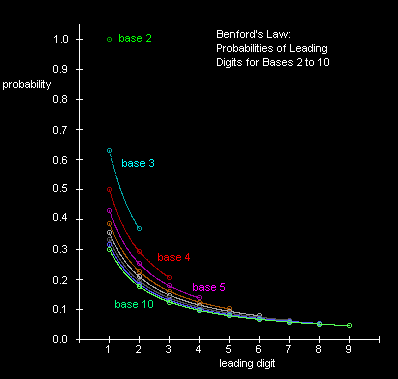## This is a mirror of this document.

I'm mirroring it because the other document may randomly go away someday, and I find the information too useful.

## This document uses MathML.

If, as a consequence, the equations don't look right to you, try looking at this image that shows what the properly rendered equations would look like.

## Benford's Law

Benford's Law (which was first stated by Simon Newcomb in 1881) states that if you randomly select a number from a table of physical constants or statistical data, the probability that the first digit will be a "1" is about 0.301, rather than 0.1 as we might expect if all digits were equally likely. In general, the "law" says that the probability of the first digit being a "d" is

${\mathrm{log}}_{10}\left(1+\frac{1}{d}\right)$

This implies that a number in a table of physical constants is more likely to begin with a smaller digit than a larger digit. It was published by Newcomb in a paper entitled "Note on the Frequency of Use of the Different Digits in Natural Numbers", which appeared in The American Journal of Mathematics (1881) 4, 39-40. It was re-discovered by Benford in 1938, and he published an article called "The Law of Anomalous Numbers" in Proc. Amer. Phil. Soc 78, pp 551-72.

Just for fun, I tabulated the first-digits of the physical constants listed in Table 2.3 of Abramowitz and Stegun's "Handbook of Mathematical Functions":

```      1
1
1*
1
1
1  2
1  2
1  2*
1  2
1  2   *
1  2     4  5
1  2     4* 5
1  2     4  5* 6        9
1  2     4  5  6   *    9*
1  2  3  4  5  6  7  8  9```

The "*" symbols indicate the approximate predicted number of occurrances according to Benford's Law. Aside from the conspicuous deficiency of 3's, that's not a bad match for just 44 data points.

Although there have been many lengthy "explanations" for Benford's Law, it seems to me this is a good candidate for a "Proof Without Words":

`   1---------------2---------3-------4-----5----6---7--8--9`

[Okay, not entirely without words:] The underlying premise is simply that physical constants, expressed in the base 10 and more or less arbitrary units, will be somewhat evenly distributed on a logarithmic scale. This is confirmed by the fact that the exponents on these constants are fairly uniformly distributed, at least over several "decades". As a result, the probability of the leading digit being "d" clearly approaches

$\frac{{\mathrm{log}}_{10}\left(d+1\right)-{\mathrm{log}}_{10}d}{{\mathrm{log}}_{10}10-{\mathrm{log}}_{10}1}={\mathrm{log}}_{10}\left(1+\frac{1}{d}\right)$

Of course, we COULD have chosen units for our physical constants such that the leading digits were all 9's (for example), but evidently we have a natural tendancy to choose units so that our numbers are evenly distributed by order of magnitude, rather than absolute value. This may be related to our basic impressions of hearing and sight (not to mention earthquakes), where our intuitive senses of loudness and brightness are logarithmic.

Naturally we can apply Benford's Law to numbers expressed in any base, not just the base 10. In general the probability of the leading digit d (in the range 1 to b-1) for the base b is

$\mathrm{Pr}\left\{d\right\}=\frac{\mathrm{ln}\left(1+\frac{1}{d}\right)}{\mathrm{ln}\left(b\right)}$

Notice that for binary numbers, i.e., numbers expressed in the base 2, the probability of the leading digit being 1 is 1.000, as it must be, since the leading digit of a binary number is necessarily 1. The distributions of probabilities of the digits 1 to b-1 for each base b from 2 to 10 are shown belowWe can also easily verify that the sum of all the probabilities for digits 1 through b-1 equals 1.0000, as it must, since the leading digit must be one of these. This implies

$\mathrm{ln}\left(1+\frac{1}{1}\right)+\mathrm{ln}\left(1+\frac{1}{2}\right)+\mathrm{. . .}+\mathrm{ln}\left(1+\frac{1}{b-1}\right)=\mathrm{ln}\left(b\right)$

By the law of logarithms, $\mathrm{ln}\left(ab\right)=\mathrm{ln}\left(a\right)+\mathrm{ln}\left(b\right)$ , this is equivalent to

$\mathrm{ln}\left(\frac{2}{1}*\frac{3}{2}*\frac{4}{3}*\mathrm{. . .}*\frac{b}{b-1}\right)=\mathrm{ln}\left(b\right)$

which confirms the result.